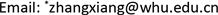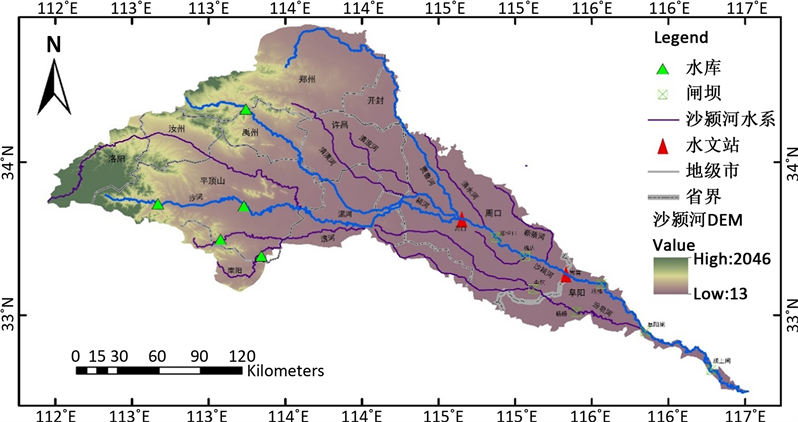﻿ 基于熵权的模糊物元模型在河流健康评价中的应用研究 Application of Fuzzy Matter-Element Model Based on Entropy Weight in River Health Assessment

Journal of Water Resources Research
Vol. 09  No. 04 ( 2020 ), Article ID: 37222 , 9 pages
10.12677/JWRR.2020.94041

Application of Fuzzy Matter-Element Model Based on Entropy Weight in River Health Assessment

Marvin C. Thompson1,2, Xiang Zhang1,2*, Liangkun Deng1,2, Jing Xu1,2, Yi Xiao1,2

1State Key Laboratory of Water Resources and Hydropower Engineering Science, Wuhan University, Wuhan Hubei

2Hubei Key Lab of Water System Science for Sponge City Construction, Wuhan HubeiReceived: Aug. 3rd, 2020; accepted: Aug. 18th, 2020; published: Aug. 25th, 2020ABSTRACT

In recent years, the health status of rivers has been deteriorating under the influence of human development, and reasonable river health assessment has gradually become the most important part of river management. However, the health assessment of highly regulated rivers considering both natural and social attributes is rarely carried out. In this paper, a new river health evaluation indicator system is constructed, which integrated four aspects of hydrology, water quality, water ecology and social services. The evaluation grades are divided into five grades, including I (ideal condition), II (health), III (sub-health), IV (unhealth), V (serious illness). The Zhoukou, Jieshou and Yingshang sections at Shaying River are selected to be evaluated using the fuzzy matter-element model based on entropy weight. According to the evaluation results, the three sections are all in sub-health condition. Although they are in better health conditions compared to the rivers in grade IV and V, the river management of Shaying River still needs to be carried out continuously. In addition, it also compares the importance of each selected indicator and discusses the necessity of incorporating the river social attribute into the indicator system by setting different scenarios. It is concluded that it is very necessary to consider the social service indicator. The research results of this paper have important reference values for more comprehensive evaluation of river health status and decision making of river health protection measures.

Keywords:Fuzzy Matter-Element Model, River Health Assessment, Shaying RiverMarvin C. Thompson1,2，张翔1,2*，邓梁堃1,2，徐晶1,2，肖宜1,2

1武汉大学水资源与水电工程科学国家重点实验室，湖北 武汉

2海绵城市建设水系统科学湖北省重点实验室，湖北 武汉1. 引言

2. 研究方法

2.1. 评价体系

2.1.1. 评价体系指标

1) 水文水资源

${\alpha }_{ij}=\frac{{D}_{ij}}{D}=\frac{\sum \mathrm{sgn}\left({Q}_{ijk}-{Q}_{ij}\right)}{D}$ (1)

$\mathrm{sgn}\left({Q}_{ijk}-{Q}_{ij}\right)=\left\{\begin{array}{l}1\text{\hspace{0.17em}}\text{\hspace{0.17em}}\text{\hspace{0.17em}}{Q}_{ijk}>{Q}_{ij}\hfill \\ 0\text{\hspace{0.17em}}\text{\hspace{0.17em}}\text{\hspace{0.17em}}{Q}_{ijk}<{Q}_{ij}\hfill \end{array}$ (2)

2) 水质Table 1. The score distribution of organic pollutionTable 2. The score distribution of heavy metal pollution

3) 水生态

$H=-\underset{i=1}{\overset{s}{\sum }}\left(pi\right)\left(\mathrm{ln}pi\right)$ (3)

4) 社会服务

${\beta }_{ij}=\frac{{D}_{ij}}{D}=\frac{\sum \mathrm{sgn}\left({Z}_{ijk}-{Z}_{ij}\right)}{D}$ (4)

$\mathrm{sgn}\left({Z}_{ijk}-{Z}_{ij}\right)=\left\{\begin{array}{l}1\text{\hspace{0.17em}}\text{\hspace{0.17em}}\text{\hspace{0.17em}}{Z}_{ijk}>{Z}_{ij}\hfill \\ 0\text{\hspace{0.17em}}\text{\hspace{0.17em}}\text{\hspace{0.17em}}{Z}_{ijk}<{Z}_{ij}\hfill \end{array}$ (5)

${R}_{u}=\frac{{W}_{u}}{{W}_{t}}$ (6)

2.1.2. 评价体系建立Table 3. River health comprehensive evaluation system

2.1.3. 评价标准

2.2. 模糊物元模型

2.2.1. 模糊物元

${R}_{nm}=\left[\begin{array}{cccc}& {M}_{1}& \cdots & {M}_{m}\\ {C}_{1}& {v}_{11}& \cdots & {v}_{1m}\\ ⋮& ⋮& & ⋮\\ {C}_{n}& {v}_{n1}& \cdots & {v}_{nm}\end{array}\right]$ (7)

2.2.2. 从优隶属度模糊物元

${\stackrel{˜}{R}}_{nm}=\left[\begin{array}{cccc}& {M}_{1}& \cdots & {M}_{m}\\ {C}_{1}& {x}_{11}& \cdots & {x}_{1m}\\ ⋮& ⋮& & ⋮\\ {C}_{n}& {x}_{n1}& \cdots & {x}_{nm}\end{array}\right]$ (8)

2.3. 熵权法确定指标权重

3. 实例研究

3.1. 研究区域概况

3.2. 评价模型建立

3.2.1. 指标数据计算

3.2.2. 熵值法确定指标权重Figure 1. Sketch map of the Shaying River basinTable 5. Weight values of each evaluation indicator

3.2.3. 计算关联度

${R}_{\xi }=\left(\begin{array}{cccccccc}周口& 界首& 颍上& \text{I}& \text{II}& \text{III}& \text{IV}& \text{V}\\ \text{0}\text{.6779}& \text{0}\text{.6603}& \text{0}\text{.6587}& \text{0}\text{.9685}& \text{0}\text{.7275}& \text{0}\text{.5070}& \text{0}\text{.2703}& \text{0}\text{.0000}\end{array}\right)$ (9)

3.3. 结果和讨论

3.3.1. 指标的建立

3.3.2. 各指标的重要程度

${R}_{\xi }=\left(\begin{array}{cccccccc}周口& 界首& 颍上& \text{I}& \text{II}& \text{III}& \text{IV}& \text{V}\\ \text{0}\text{.6327}& \text{0}\text{.5408}& \text{0}\text{.4891}& \text{0}\text{.9670}& \text{0}\text{.7266}& \text{0}\text{.4936}& \text{0}\text{.2330}& \text{0}\text{.0000}\end{array}\right)$ (10)

4. 结论

Marvin C. Thompson,张 翔,邓梁堃,徐 晶,肖 宜. 基于熵权的模糊物元模型在河流健康评价中的应用研究
Application of Fuzzy Matter-Element Model Based on Entropy Weight in River Health Assessment[J]. 水资源研究, 2020, 09(04): 394-402. https://doi.org/10.12677/JWRR.2020.94041

1. 1. SUNDARAY, S. K., PANDA, U. C., NAYAK, B. B., et al. Multivariate statistical techniques for the evaluation of spatial and temporal variations in water quality of the Mahanadi River estuarine system (India): A case study. Environmental Geochemistry and Health, 2006, 28(4): 317-330. https://doi.org/10.1007/s10653-005-9001-5

2. 2. ZHAO, Y. W., YANG, Z. F. Integra-tive fuzzy hierarchical model for river health assessment: A case study of Yong River in Ningbo City, China. Communications in Nonlinear Science and Numerical Simulation, 2009, 14(4): 1729-1736. https://doi.org/10.1016/j.cnsns.2007.09.019

3. 3. 秦鹏, 王英华, 王维汉, 等. 河流健康评价的模糊层次与可变模糊集耦合模型[J]. 浙江大学学报(工学版), 2011, 45(12): 2169-2175. QIN Peng, WANG Yinghua, WANG Weihan, et al. Integrated model of fuzzy analytical hierarchy process and variable fuzzy set model on evaluating river health system. Journal of Zhejiang University (Engineering Science), 2011, 45(12): 2169-2175. (in Chinese)

4. 4. 崔东文. RBF与GRNN神经网络模型在河流健康评价中的应用——以文山州区域中小河流健康评价为例[J]. 中国农村水利水电, 2012(3): 61-66. CUI Dongwen. RBF and GRNN neural network model in the evaluation of river health. China Rural Water and Hydropower, 2012(3): 61-66. (in Chinese)

5. 5. 邓晓军, 翟禄新, 李艺. 基于熵权模糊综合评价模型的桂林市桃花江河流健康评价[J]. 水电能源科学, 2013, 31(11): 35-38. DENG Xiaojun, ZHAI Luxin and LI Yi. Taohuajiang river health evaluation in Guilin city based on entropy weight and fuzzy comprehensive evaluation model. Water Resources and Power, 2013, 31(11): 35-38. (in Chinese)

6. 6. DENG, X., XU, Y., HAN, L., et al. Assessment of river health based on an improved entropy-based fuzzy matter-element model in the Taihu Plain, China. Ecological Indicators, 2015, 57: 85-95. https://doi.org/10.1016/j.ecolind.2015.04.020

7. 7. PAN, G., XU, Y., YU, Z., et al. Analysis of river health variation under the background of urbanization based on entropy weight and matter-element model: A case study in Huzhou City in the Yangtze River Delta, China. Environmental Research, 2015, 139: 31-35. https://doi.org/10.1016/j.envres.2015.02.013

8. 8. KARR, J. R. Defining and measuring river health. Freshwater Biology, 1999, 41: 221-234. https://doi.org/10.1046/j.1365-2427.1999.00427.x

9. 9. NORRIS, R. H., THOMAS, M. C. What is river health? Freshwa-ter Biology, 1999, 41: 197-209. https://doi.org/10.1046/j.1365-2427.1999.00425.x

10. 10. ZHANG, J., WANG, K., CHEN, X., et al. Combining a fuzzy mat-ter-element model with a geographic information system in eco-environmental sensitivity and distribution of land use planning. International Journal of Environmental Research and Public Health, 2011, 8(4): 1206-1221. https://doi.org/10.3390/ijerph8041206

11. 11. 周振民, 常慧. 基于熵权的模糊物元地下水水质评价模型[J]. 中国农村水利水电, 2008(12): 45-47. ZHOU Zhenmin, CHANG Hui. Fuzzy matter-element groundwater quality evaluation model based on entropy weight. China Ru-ral Water and Hydropower, 2008(12): 45-47. (in Chinese)

12. 12. 刘倩, 董增川, 徐伟, 等. 基于模糊物元模型的滦河河流健康评价[J]. 水电能源科学, 2014, 32(9): 47-50. LIU Qian, DONG Zengchuan, XU Wei, et al. Health assessment of Luanhe River based on fuzzy matter-element model. Water Resources and Power, 2014, 32(9): 47-50. (in Chinese)

13. 13. 孙博. 基于熵权模糊物元模型的河流生态健康综合评价[J]. 人民珠江, 2015, 36(6): 95-100. SUN Bo. River ecosystem health evaluation of fuzzy matter element model based on entropy weight. Pearl River, 2015, 36(6): 95-100. (in Chinese)

14. 14. STRONG, W. L. Biased richness and evenness relationships within Shannon-Wiener index values. Ecological Indicators, 2016(67): 703-713. https://doi.org/10.1016/j.ecolind.2016.03.043

15. 15. 夏军, 石卫. 变化环境下中国水安全问题研究与展望[J]. 水利学报, 2016, 47(3): 292-301. XIA Jun, SHI Wei. Perspective on water security issue of changing environment in China. Journal of Hydraulic Engineering, 2016, 47(3): 292-301. (in Chinese)

16. 16. 葛金金, 彭文启, 张汶海, 等. 确定河道内适宜生态流量的几种水文学方法——以沙颍河周口段为例[J]. 南水北调与水利科技, 2019, 17(2): 75-80. GE Jinjin, PENG Wenqi, ZHANG Wenhai, et al. Comparison of several hydrological methods to determine the ecological flow for instream river: A case study of Zhoukou hydrological station in Shaying River. South-to-North Water Transfers and Water Science & Technology, 2019, 17(2): 75-80. (in Chinese)

17. 17. ZUO, Q. T., JIN, R. F., MA, J. X., et al. Description and applica-tion of a mathematical method for the analysis of harmony. The Scientific World Journal, 2015, 2015: Article ID: 831396. https://doi.org/10.1155/2015/831396